# Civil Engineering - RCC Structures Design

### Exercise :: RCC Structures Design - Section 5

46.

If T and R are the tread and rise of a stair which carries a load w per square metre on slope, the corresponding load per square metre of the horizontal area, is

 A.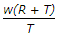B.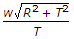C.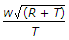D.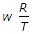E.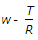Explanation:

No answer description available for this question. Let us discuss.

47.

If the bearing capacity of soil is 10 tonnes/cm2 and the projection of plain concrete footing from walls, is a cm, the depth D of footing is

 A. D = 0.0775 a B. D = 0.775 a C. D = 0.775a D. D = 0.775 a2

Explanation:

No answer description available for this question. Let us discuss.

48.

An R.C.C. lintel is spanning an opening of 2 m span in a brick wall. The height of the roof is 2.9 m above the floor level and that of the opening is 2.1 m above the floor level. The lintel is to be designed for self weight plus

 A. triangular load of the wall B. UDL of wall C. UDL of wall + load from the roof D. triangular load + load from the roof.

Explanation:

No answer description available for this question. Let us discuss.

49.

The pitch of the main bars in a simply supported slab, should not exceed its effective depth by

 A. three times B. four times C. five times D. six times E. seven times.